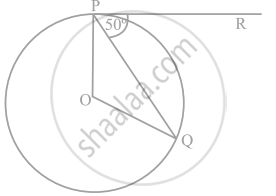# In the following figure, if O is the centre of a circle, PQ is a chord and the tangent PR at P makes an angle of 50° with PQ, then ∠POQ is equal to - Mathematics

MCQ
Fill in the Blanks

In the following figure, if O is the centre of a circle, PQ is a chord and the tangent PR at P makes an angle of 50° with PQ, then ∠POQ is equal to ______• 100°

• 80°

• 90°

• 75°

#### Solution

In the following figure, if O is the centre of a circle, PQ is a chord and the tangent PR at P makes an angle of 50° with PQ, then ∠POQ is equal to 100°.

Explanation:

From figure using properties of circle and tangents

∠OPQ = 90° - 50°

∠OPQ = 40°

So (∠E) = ∠OQP = 40°

Now In ∆POQ

∠POQ = 180° – (∠OPQ + ∠OQP)

∠POQ = 180° – (40° + 40°)

∠POQ = 100°

Concept: Concept of Circle - Centre, Radius, Diameter, Arc, Sector, Chord, Segment, Semicircle, Circumference, Interior and Exterior, Concentric Circles
Is there an error in this question or solution?

#### APPEARS IN

NCERT Mathematics Exemplar Class 10
Chapter 9 Circles
Exercise 9.1 | Q 7 | Page 103
Share